# K-Nearest Neighbor(KNN) algorithm in Machine Learning

## Contents

• Introduction
• How does KNN work?
• Euclidean Distance
• Manhattan Distance
• KNN with the imbalanced dataset.
• Lazy Learners.

K-nearest neighbor (KNN) is one of the most important supervised machine learning algorithms. It is used for both classifications as well as a regression problem. It is used to classify the non-linear data points that means if your data points are not distributed in a linear way you can not draw a straight line to classify the data points. In such a scenario, we mostly use the Knn algorithm. It is mostly used for classification problems.

## How do the KNN algorithm works?

Now let’s take a deep dive and see how does this KNN algorithm works?

We will try to understand this algorithm with the help of the classification problem. Let suppose we have some data points representing two different categories, red star(category one) and green triangle(category two).

What happened when a new data point is introduced in it? How does the algorithm will decide which category does it belong to? So let's find out the answers to these questions. Let suppose that a new data point is introduced.

Here, the k value will come into the pictures. so How do we decide whether these new data points belong to category one or category two? This will be decided with the help of the ‘K’ value. What is this k value represent here? The k value is nothing but the number of the nearest neighbor.

Let’s suppose that I have chosen the k value as k = 5. This means that we need to find the 5 nearest points as shown in the figure below.

If you look at the figure carefully you will find that there are 3 points from the red and 2 points from the green which are closer to the new points. How did we find these nearest points?

We calculated the distance of each nearest neighbor. There are two methods to calculate the distances.

1. Euclidian Distance
2. Manhattan Distance

## Euclidian Distance

In mathematics terms, euclidian distance is the distance between two points. It is calculated based on the Pythagoras theorem. Let’s see how euclidian distance is calculated? consider two points in a plane say p(x1,y1) and q(x2,y2). The formula is derived as shown in the figure.

## Manhattan Distance

Manhattan distance between two points is defined as the sum of the magnitude of the distance in the n-dimension. Its formula is given as follows:

Now let us come to our KNN algorithm. So if you see the new data points. if you notice, the maximum number of neighbors are from category one i.e. the red points, So we conclude that the new data points belong to category one.

## What happens if the dataset is imbalanced?

So here is a question arises that what will happen if the dataset is imbalanced? Will the KNN be biased concerning output? Let's see the answers to all these questions.

Consider an imbalanced dataset. Let us suppose that this dataset contains 1000 “Yes” and 100 “NO”. Now let us suppose that new data points are introduced there. So here is the question which will come to your mind. To which category do these new points will belong? will it be biased towards category one which contains 1000 “Yes”? So the answer to this question is yes. It is definitely going to be biased towards the “Yes” category. Let suppose, if I choose the k value as 100 and now try to find out the nearest point. Then most of the neighbors will be from the category “Yes”. There will be very few neighbors from category “NO”.

Note: K-nearest neighbors are affected by the outliers.

## Why KNN algorithm is called a Lazy Learner?

This is a very interesting topic in KNN. So why do we called KNN a lazy learner? The reason for this is that, when you provide training data to the KNN algorithm, it does not perform the training on that data, instead it stores the dataset. In other words, there is no training time present there. It does not perform any calculation at this point. So when does the computation really start?

So it does the computation at the time prediction when it gets the new data points. The prediction steps in the KNN model are very expensive because whenever you will make a prediction you need to find the nearest neighbor in the whole training dataset and then perform prediction. This is the reason it is called a lazy learner.

• KNN algorithm is very simple and easy to implement.
• It can work easily with multiclass datasets.
• It requires no training phase. This is the reason it is also called the lazy learner as explained above.
• We can easily add the additional training data to the model. The additional data does not require retraining the model.
• One of the best advantages of KNN is that we can use it for regression as well as classification problems.
• It does not require any additional assumptions due to its non-parametric nature.

• It is considered a very slow algorithm when it comes to making predictions.
• It is difficult to find the optimal value of K.
• It is very sensitive to the outliers because it uses the distance criteria.
• It is sensitive to the dimensions. As the number of dimensions increases, it becomes hard for the algorithm to make the prediction. Calculating the distance becomes difficult.
• It requires feature scaling.

So we have seen the whole intuition about the KNN algorithm. We saw that KNN is a very simple and easy-to-use algorithm for regression as well as classification problems. We also saw that why is it called the lazy learner algorithm. We can use the KNN algorithm in many use cases such as detecting the patterns in credit cards, diabetes prediction, we also use it for building a recommendation system if data is not very large.

--

--

--

## More from Aditya Kumar Pandey

Love podcasts or audiobooks? Learn on the go with our new app.

## Unsupervised Learning with k-means part 1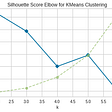## Do even the best DNN models understand language?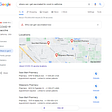## Hyper-parameter tuning for scikit-learn ML models## A Hebbian cell assembly is formed at full strength on a single trial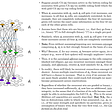## Training a Core ML Model for Sentiment Analysis## Why Deep Learning is Now Easy for Data Scientists |## Machine Learing : K-Nearest Neighbors (Theory Explained)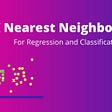## ML algorithm on DNA sequences of Disease## Accuracy — Performance prediction of the model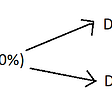## OneHotEncoder in one go